### Home > PC > Chapter 4 > Lesson 4.2.4 > Problem4-111

4-111.
1. It is sometimes useful to convert a trig expression into one that has only one trigonometric function. This can be done by first changing all of the terms to sine or cosine and utilizing the Pythagorean identities. Express each of the following using only sin A. Homework Help ✎

1. tan A · sec A

2. (tan2 A)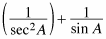3. (1 + cot2 A) csc2 A

4.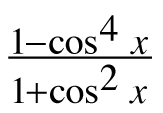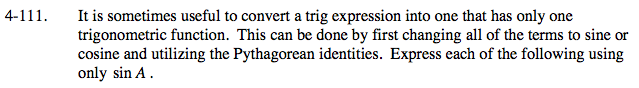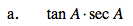1. Change all of the terms to sine or cosine.
2. Simplify.
3. Substitute using the Fundamental Pythagorean Identities.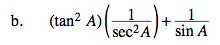1. Change all terms to sine and cosine.
2. Simplify
3. Get a common denominator.

$\frac{\text{sin}^3A+1}{\text{sin}A}$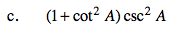1. Substitute using a Fundamental Pythagorean Identity.
2. Simplify.
3. Change terms to sine.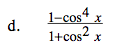1. Factor the numerator.
2. Simplify.
3. Substitute using the Fundamental Pythagorean Identities.